# How to Calculate and Solve for Permeability | Mass Transfer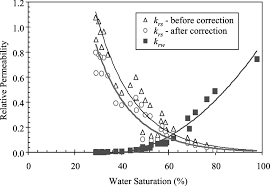The image above represents permeability.

To compute for permeability, five essential parameters are needed and these parameters are Constant (A), Partial Pressure (Po), Activation due to Permeation (Qp), Gas Constant (R) and Temperature (T).

The formula for calculating permeability:

P = APo0.5 . e-Qp/RT

Where:

P = Permeability
A = Constant
Po = Partial Pressure
Qp = Activation due to Permeation
R = Gas Constant
T = Temperature

Let’s solve an example;
Find the permeability when the constant is 12, the partial pressure is 22, the activation due to permeation is 10, the gas constant is 14 and the temperature is 2.

This implies that;

A = Constant = 12
Po = Partial Pressure = 22
Qp = Activation due to Permeation = 10
R = Gas Constant = 14
T = Temperature = 2

P = APo0.5 . e-Qp/RT
P = 12(22)0.5 . e-(10)/(14)(2)
P = 12(4.69) . e-10/28
P = 56.28 . e-0.357
P = 56.28 (0.699)
P = 39.38

Therefore, the permeability is 39.38.

Nickzom Calculator – The Calculator Encyclopedia is capable of calculating the permeability.

To get the answer and workings of the permeability using the Nickzom Calculator – The Calculator Encyclopedia. First, you need to obtain the app.

You can get this app via any of these means:

To get access to the professional version via web, you need to register and subscribe for NGN 2,000 per annum to have utter access to all functionalities.
You can also try the demo version via https://www.nickzom.org/calculator

Apple (Paid) – https://itunes.apple.com/us/app/nickzom-calculator/id1331162702?mt=8
Once, you have obtained the calculator encyclopedia app, proceed to the Calculator Map, then click on Materials and Metallurgical under Engineering.Now, Click on Mass Transport under Materials and Metallurgical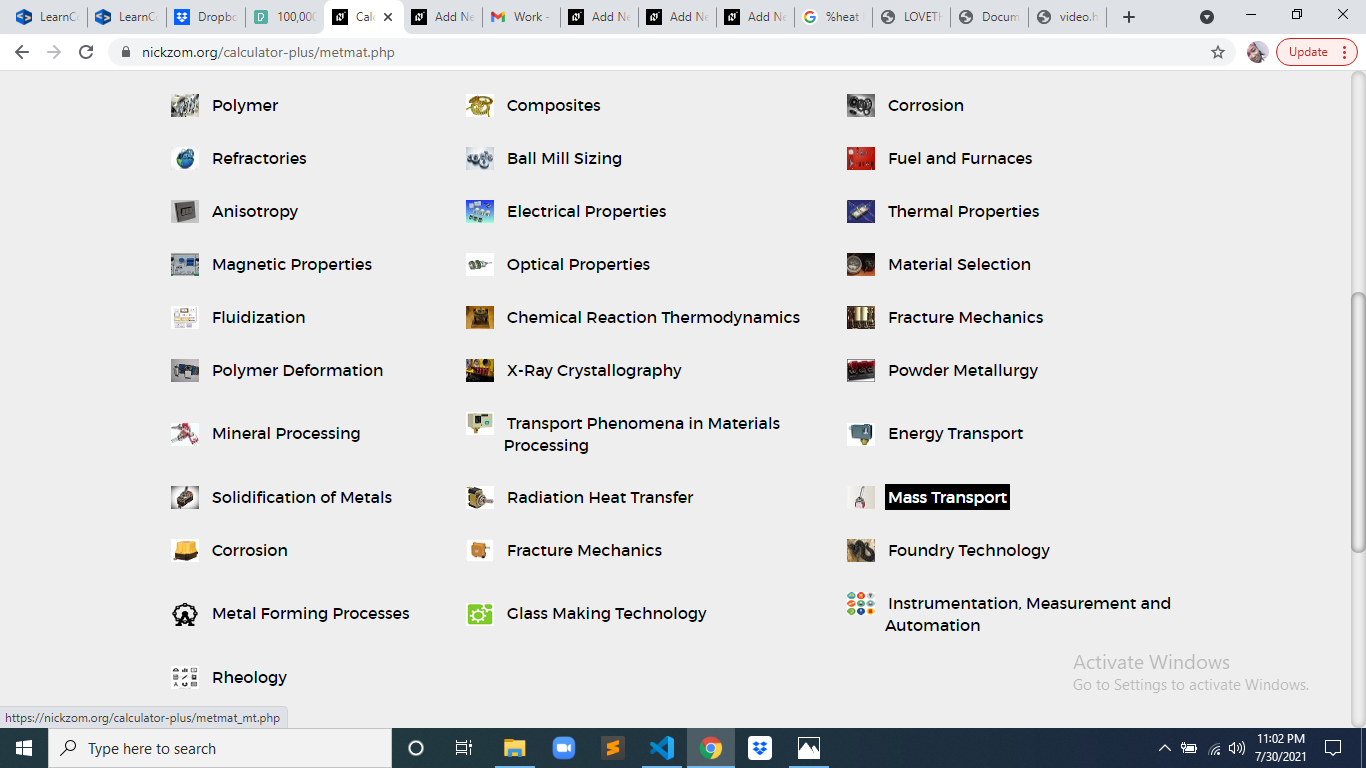Now, Click on Permeability under Mass Transport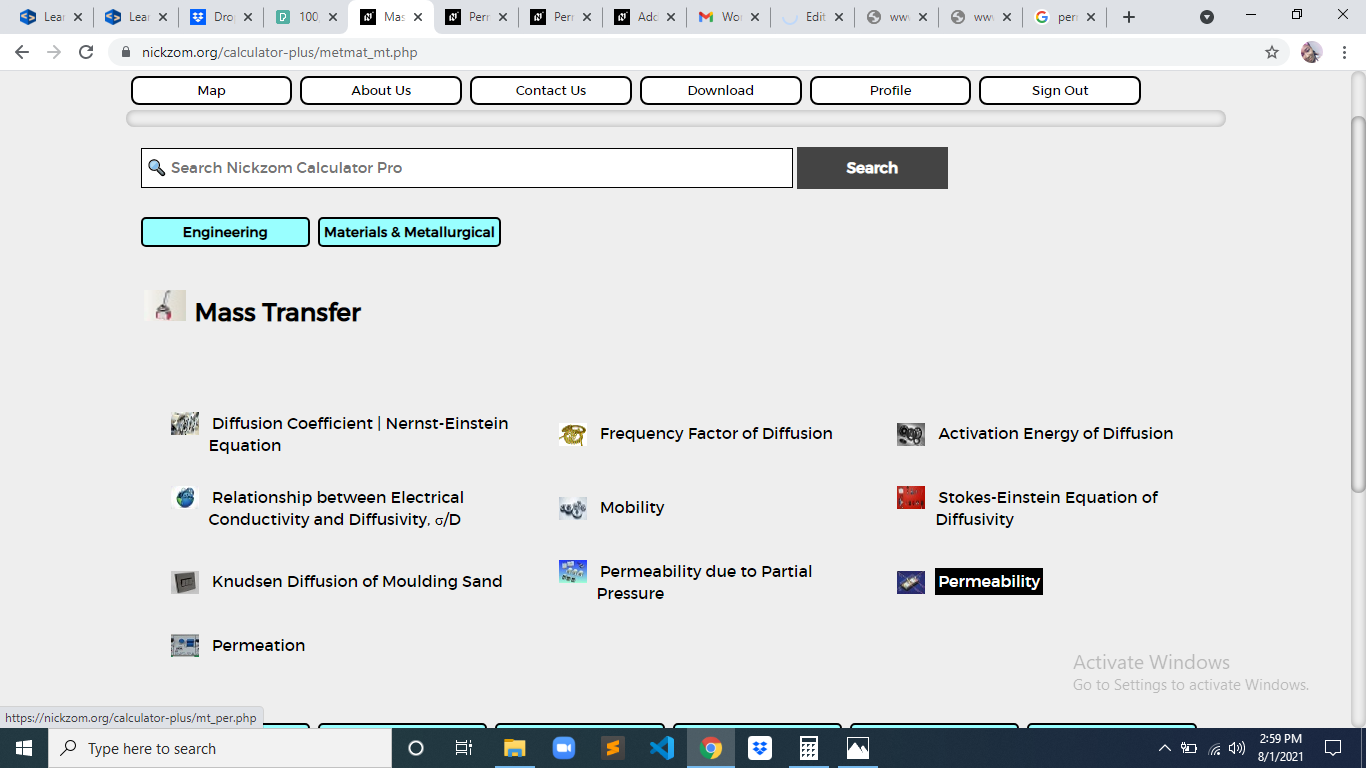The screenshot below displays the page or activity to enter your values, to get the answer for the permeability according to the respective parameter which is the Constant (A), Partial Pressure (Po), Activation due to Permeation (Qp), Gas Constant (R) and Temperature (T).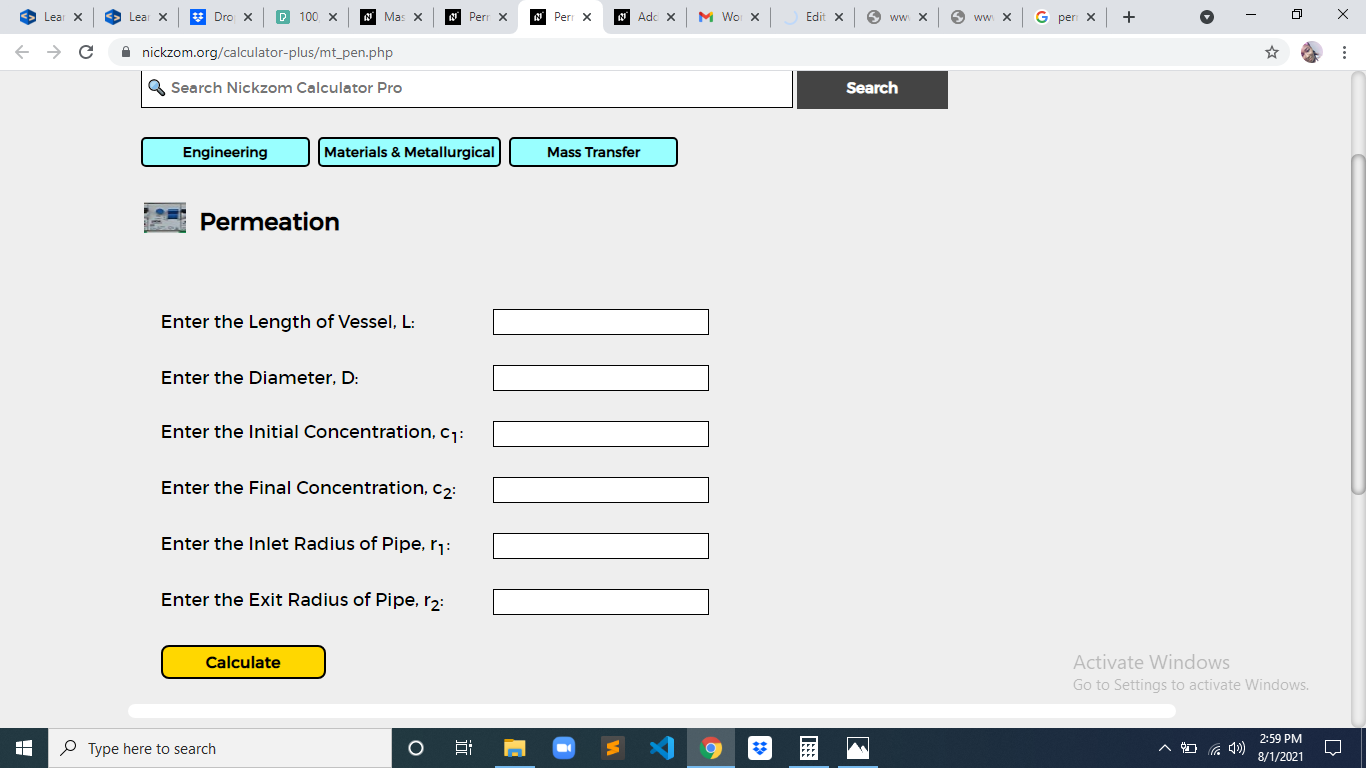Now, enter the values appropriately and accordingly for the parameters as required by the Constant (A) is 12, Partial Pressure (Po) is 22, Activation due to Permeation (Qp) is 10, Gas Constant (R) is 14 and Temperature (T) is 2.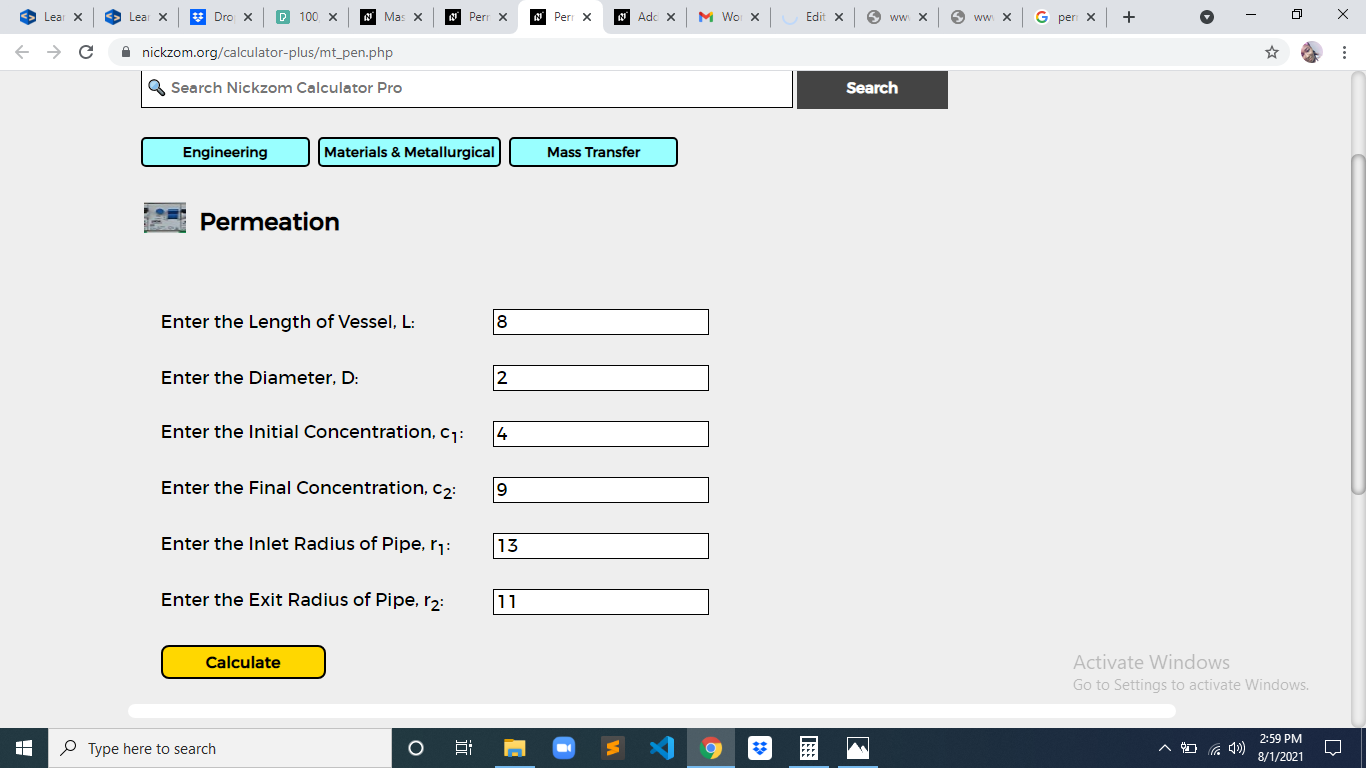Finally, Click on Calculate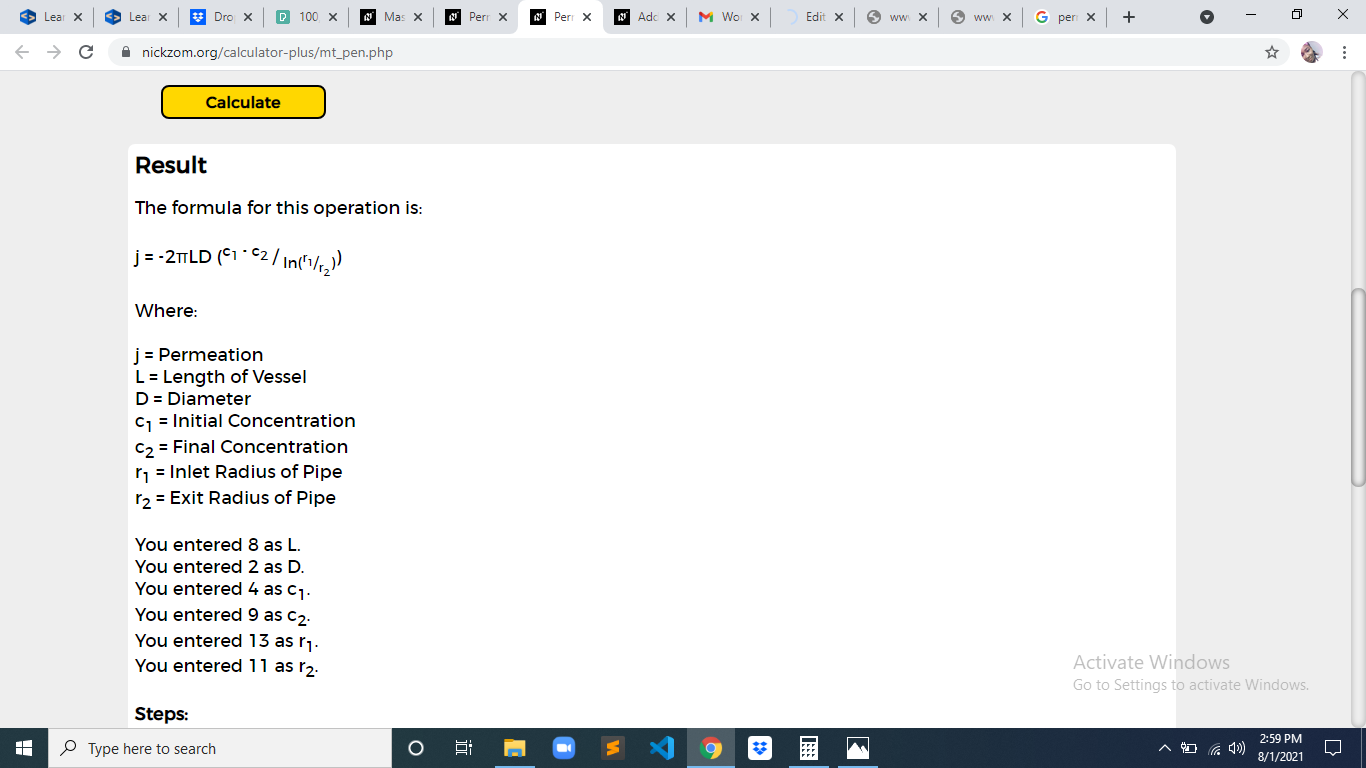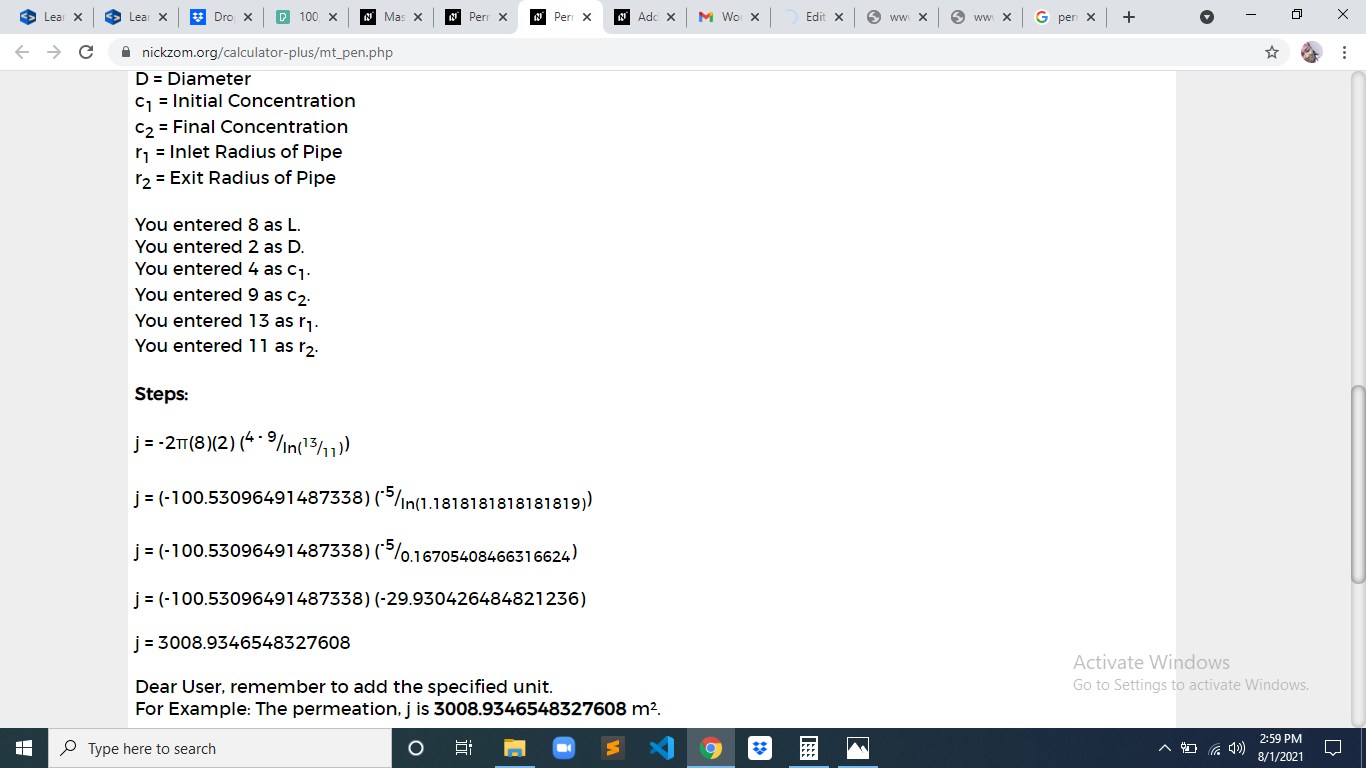As you can see from the screenshot above, Nickzom Calculator– The Calculator Encyclopedia solves for the permeability and presents the formula, workings and steps too.